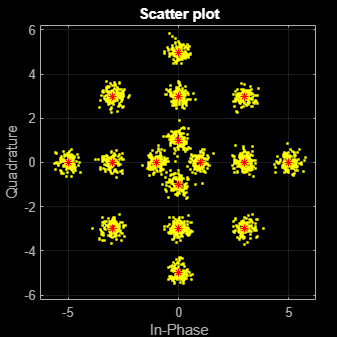genqammod

Description

example

y = genqammod(x,const) returns the complex envelop of the QAM for message signal x. Input const specifies the signal mapping for the modulation.

Examples

collapse all

Transmit and receive data using a nonrectangular 16-ary constellation in the presence of Gaussian noise. Show the scatter plot of the noisy constellation and estimate the symbol error rate (SER) for two different signal-to-noise ratios.

Create a 16-QAM constellation based on the V.29 standard for telephone-line modems.

c = [-5 -5i 5 5i -3 -3-3i -3i 3-3i 3 3+3i 3i -3+3i -1 -1i 1 1i];
M = length(c);

Generate random symbols.

data = randi([0 M-1],2000,1);

Modulate the data by using the genqammod function. General QAM modulation is necessary because the custom constellation is not rectangular.

modData = genqammod(data,c);

Pass the signal through an AWGN channel having a 20 dB signal-to-noise ratio (SNR).

rxSig = awgn(modData,20,'measured');

Display a scatter plot of the received signal and the reference constellation, c.

h = scatterplot(rxSig);
hold on
scatterplot(c,[],[],'r*',h)
grid
hold offDemodulate the received signal by using the genqamdemod function. Determine the number of symbol errors and the symbol error ratio.

demodData = genqamdemod(rxSig,c);
[numErrors,ser] = symerr(data,demodData)
numErrors = 1
ser = 5.0000e-04

Repeat the transmission and demodulation process with an AWGN channel having a 10 dB SNR. Determine the symbol error rate for the reduced SNR. As expected, the performance degrades when the SNR is decreased.

rxSig = awgn(modData,10,'measured');
demodData = genqamdemod(rxSig,c);
[numErrors,ser] = symerr(data,demodData)
numErrors = 462
ser = 0.2310

Create the points that describe a hexagonal constellation.

inphase = [1/2 1 1 1/2 1/2 2 2 5/2];
quadr = [0 1 -1 2 -2 1 -1 0];
inphase = [inphase;-inphase]; inphase = inphase(:);

Plot the constellation.

h = scatterplot(const);Generate input data symbols. Modulate the symbols using this constellation.

x = [3 8 5 10 7];
y = genqammod(x,const);

Demodulate the modulated signal, y.

z = genqamdemod(y,const);

Plot the modulated signal in same figure.

hold on;
scatterplot(y,1,0,'ro',h);
legend('Constellation','Modulated signal');Determine the number of symbol errors between the demodulated data to the original sequence.

numErrs = symerr(x,z)
numErrs = 0

Input Arguments

collapse all

Message signal, specified as a scalar, vector, matrix, or 3-D array of numeric values. The message signal must consist of integers from 0 and length(const)–1. If x is a matrix with multiple rows, the function processes the columns independently.

Data Types: double | single | fi | int8 | int16 | uint8 | uint16

Signal mapping, specified as a complex vector.

Data Types: double | single | fi | int8 | int16 | uint8 | uint16
Complex Number Support: Yes

Output Arguments

collapse all

Complex envelope, returned as a scalar, vector, matrix, or 3-D array of numeric values. The length of y is the same as the length of input x.

Data Types: double | single | fi | int8 | int16 | uint8 | uint16